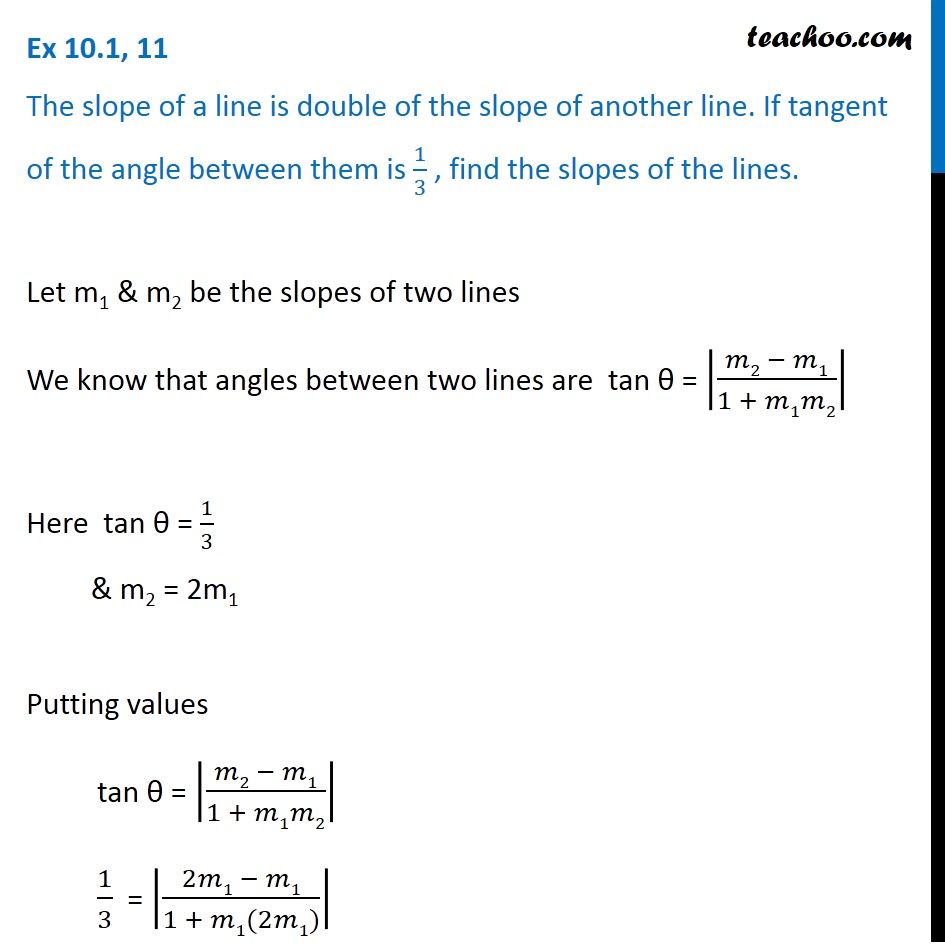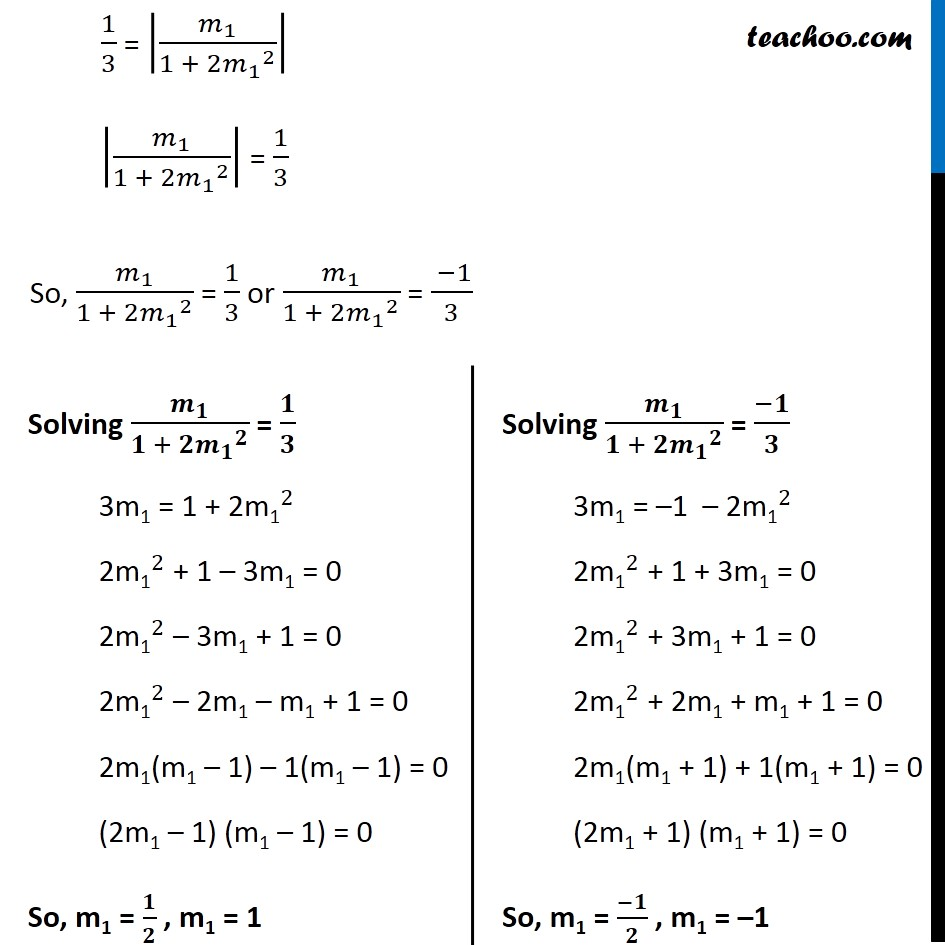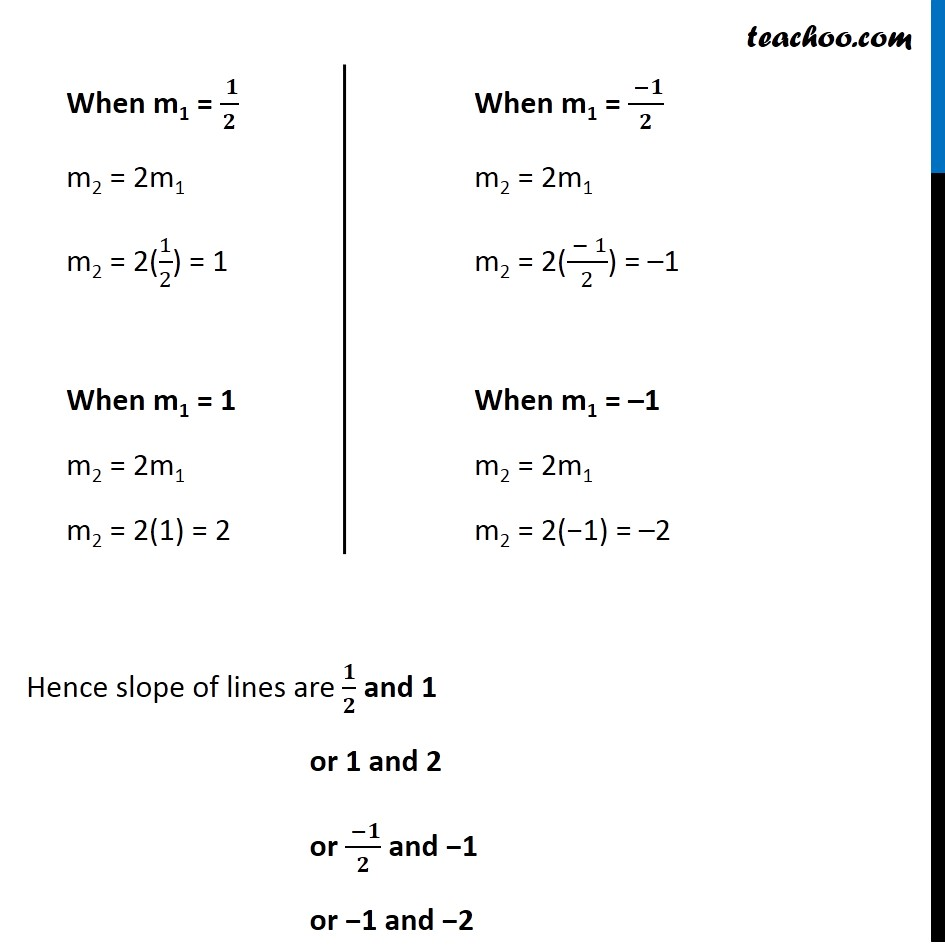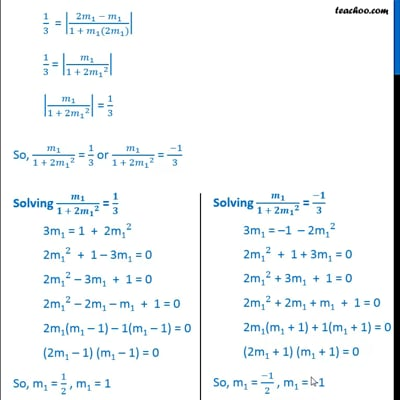Ex 10.1

Chapter 10 Class 11 Straight Lines
Serial order wiseThis video is only available for Teachoo black users

Solve all your doubts with Teachoo Black (new monthly pack available now!)

### Transcript

Ex 10.1, 11 The slope of a line is double of the slope of another line. If tangent of the angle between them is 1/3 , find the slopes of the lines. Let m1 & m2 be the slopes of two lines We know that angles between two lines are tan θ = |(𝑚2 − 𝑚1)/(1 + 𝑚1𝑚2)| Here tan θ = 1/3 & m2 = 2m1 Putting values tan θ = |(𝑚2 − 𝑚1)/(1 + 𝑚1𝑚2)| 1/3 = |(2𝑚1 − 𝑚1)/(1 + 𝑚1(2𝑚1))| 1/3 = |𝑚_1/(1 + 2〖𝑚_1〗^2 )| |𝑚_1/(1 + 2〖𝑚_1〗^2 )| = 1/3 So, 𝑚_1/(1 + 2〖𝑚_1〗^2 ) = 1/3 or 𝑚_1/(1 + 2〖𝑚_1〗^2 ) = ( −1)/3 Solving 𝒎_𝟏/(𝟏 + 𝟐〖𝒎_𝟏〗^𝟐 ) = 𝟏/𝟑 3m1 = 1 + 2〖"m1" 〗^2 2〖"m1" 〗^2 + 1 – 3m1 = 0 2〖"m1" 〗^2 – 3m1 + 1 = 0 2〖"m1" 〗^2 – 2m1 – m1 + 1 = 0 2m1(m1 – 1) – 1(m1 – 1) = 0 (2m1 – 1) (m1 – 1) = 0 So, m1 = 𝟏/𝟐 , m1 = 1 Solving 𝒎_𝟏/(𝟏 + 𝟐〖𝒎_𝟏〗^𝟐 ) = (−𝟏)/𝟑 3m1 = –1 – 2〖"m1" 〗^2 2〖"m1" 〗^2 + 1 + 3m1 = 0 2〖"m1" 〗^2 + 3m1 + 1 = 0 2〖"m1" 〗^2 + 2m1 + m1 + 1 = 0 2m1(m1 + 1) + 1(m1 + 1) = 0 (2m1 + 1) (m1 + 1) = 0 So, m1 = (−𝟏)/𝟐 , m1 = –1 When m1 = ( 𝟏)/𝟐 m2 = 2m1 m2 = 2(1/2) = 1 When m1 = 1 m2 = 2m1 m2 = 2(1) = 2 When m1 = ( −𝟏)/𝟐 m2 = 2m1 m2 = 2(( − 1)/2) = –1 When m1 = –1 m2 = 2m1 m2 = 2(−1) = –2 Hence slope of lines are 𝟏/𝟐 and 1 or 1 and 2 or ( −𝟏)/𝟐 and −1 or −1 and −2